Prealgebra

# 5.2Decimal Operations

Prealgebra5.2 Decimal Operations

### Learning Objectives

By the end of this section, you will be able to:
• Multiply decimals
• Divide decimals
• Use decimals in money applications

### Be Prepared 5.2

Before you get started, take this readiness quiz.

• Simplify $70100.70100.$
If you missed this problem, review Example 4.19.
• Multiply $310·910.310·910.$
If you missed this problem, review Example 4.25.
• Divide $−36÷(−9).−36÷(−9).$
If you missed this problem, review Example 3.49.

Let’s take one more look at the lunch order from the start of Decimals, this time noticing how the numbers were added together.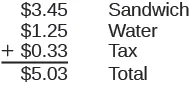All three items (sandwich, water, tax) were priced in dollars and cents, so we lined up the dollars under the dollars and the cents under the cents, with the decimal points lined up between them. Then we just added each column, as if we were adding whole numbers. By lining up decimals this way, we can add or subtract the corresponding place values just as we did with whole numbers.

### How To

1. Step 1. Write the numbers vertically so the decimal points line up.
2. Step 2. Use zeros as place holders, as needed.
3. Step 3. Add or subtract the numbers as if they were whole numbers. Then place the decimal in the answer under the decimal points in the given numbers.

### Example 5.11

Add: $3.7+12.4.3.7+12.4.$

### Try It 5.21

Add: $5.7+11.9.5.7+11.9.$

### Try It 5.22

Add: $18.32+14.79.18.32+14.79.$

### Example 5.12

Add: $23.5+41.38.23.5+41.38.$

### Try It 5.23

Add: $4.8+11.69.4.8+11.69.$

### Try It 5.24

Add: $5.123+18.47.5.123+18.47.$

How much change would you get if you handed the cashier a $2020$ bill for a $14.6514.65$ purchase? We will show the steps to calculate this in the next example.

### Example 5.13

Subtract: $20−14.65.20−14.65.$

### Try It 5.25

Subtract:

$10−9.58.10−9.58.$

### Try It 5.26

Subtract:

$50−37.42.50−37.42.$

### Example 5.14

Subtract: $2.51−7.4.2.51−7.4.$

### Try It 5.27

Subtract: $4.77−6.3.4.77−6.3.$

### Try It 5.28

Subtract: $8.12−11.7.8.12−11.7.$

### Multiply Decimals

Multiplying decimals is very much like multiplying whole numbers—we just have to determine where to place the decimal point. The procedure for multiplying decimals will make sense if we first review multiplying fractions.

Do you remember how to multiply fractions? To multiply fractions, you multiply the numerators and then multiply the denominators.

So let’s see what we would get as the product of decimals by converting them to fractions first. We will do two examples side-by-side in Table 5.3. Look for a pattern.

A B
$(0.3)(0.7)(0.3)(0.7)$ $(0.2)(0.46)(0.2)(0.46)$
Convert to fractions. $(310)(710)(310)(710)$ $(210)(46100)(210)(46100)$
Multiply. $2110021100$ $921000921000$
Convert back to decimals. $0.210.21$ $0.0920.092$
Table 5.3

There is a pattern that we can use. In A, we multiplied two numbers that each had one decimal place, and the product had two decimal places. In B, we multiplied a number with one decimal place by a number with two decimal places, and the product had three decimal places.

How many decimal places would you expect for the product of $(0.01)(0.004)?(0.01)(0.004)?$ If you said “five”, you recognized the pattern. When we multiply two numbers with decimals, we count all the decimal places in the factors—in this case two plus three—to get the number of decimal places in the product—in this case five.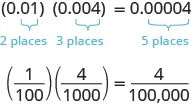Once we know how to determine the number of digits after the decimal point, we can multiply decimal numbers without converting them to fractions first. The number of decimal places in the product is the sum of the number of decimal places in the factors.

The rules for multiplying positive and negative numbers apply to decimals, too, of course.

### Multiplying Two Numbers

When multiplying two numbers,
• if their signs are the same, the product is positive.
• if their signs are different, the product is negative.

When you multiply signed decimals, first determine the sign of the product and then multiply as if the numbers were both positive. Finally, write the product with the appropriate sign.

### How To

#### Multiply decimal numbers.

1. Step 1. Determine the sign of the product.
2. Step 2. Write the numbers in vertical format, lining up the numbers on the right.
3. Step 3. Multiply the numbers as if they were whole numbers, temporarily ignoring the decimal points.
4. Step 4. Place the decimal point. The number of decimal places in the product is the sum of the number of decimal places in the factors. If needed, use zeros as placeholders.
5. Step 5. Write the product with the appropriate sign.

### Example 5.15

Multiply: $(3.9)(4.075).(3.9)(4.075).$

### Try It 5.29

Multiply: $4.5(6.107).4.5(6.107).$

### Try It 5.30

Multiply: $10.79(8.12).10.79(8.12).$

### Example 5.16

Multiply: $(−8.2)(5.19).(−8.2)(5.19).$

### Try It 5.31

Multiply: $(4.63)(−2.9).(4.63)(−2.9).$

### Try It 5.32

Multiply: $(−7.78)(4.9).(−7.78)(4.9).$

In the next example, we’ll need to add several placeholder zeros to properly place the decimal point.

### Example 5.17

Multiply: $(0.03)(0.045).(0.03)(0.045).$

### Try It 5.33

Multiply: $(0.04)(0.087).(0.04)(0.087).$

### Try It 5.34

Multiply: $(0.09)(0.067).(0.09)(0.067).$

#### Multiply by Powers of $1010$

In many fields, especially in the sciences, it is common to multiply decimals by powers of $10.10.$ Let’s see what happens when we multiply $1.94361.9436$ by some powers of $10.10.$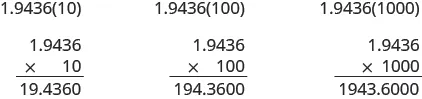Look at the results without the final zeros. Do you notice a pattern?

$1.9436(10)=19.4361.9436(100)=194.361.9436(1000)=1943.61.9436(10)=19.4361.9436(100)=194.361.9436(1000)=1943.6$

The number of places that the decimal point moved is the same as the number of zeros in the power of ten. Table 5.4 summarizes the results.

Multiply by Number of zeros Number of places decimal point moves
$1010$ $11$ $11$ place to the right
$100100$ $22$ $22$ places to the right
$1,0001,000$ $33$ $33$ places to the right
$10,00010,000$ $44$ $44$ places to the right
Table 5.4

We can use this pattern as a shortcut to multiply by powers of ten instead of multiplying using the vertical format. We can count the zeros in the power of $1010$ and then move the decimal point that same of places to the right.

So, for example, to multiply $45.8645.86$ by $100,100,$ move the decimal point $22$ places to the right.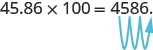Sometimes when we need to move the decimal point, there are not enough decimal places. In that case, we use zeros as placeholders. For example, let’s multiply $2.42.4$ by $100.100.$ We need to move the decimal point $22$ places to the right. Since there is only one digit to the right of the decimal point, we must write a $00$ in the hundredths place.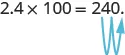### How To

#### Multiply a decimal by a power of 10.

1. Step 1. Move the decimal point to the right the same number of places as the number of zeros in the power of $10.10.$
2. Step 2. Write zeros at the end of the number as placeholders if needed.

### Example 5.18

Multiply $5.635.63$ by factors of $1010$ $100100$$1000.1000.$

### Try It 5.35

Multiply $2.582.58$ by factors of $1010$ $100100$ $1000.1000.$

### Try It 5.36

Multiply $14.214.2$ by factors of $1010$ $100100$ $1000.1000.$

### Divide Decimals

Just as with multiplication, division of decimals is very much like dividing whole numbers. We just have to figure out where the decimal point must be placed.

To understand decimal division, let’s consider the multiplication problem

$(0.2)(4)=0.8(0.2)(4)=0.8$

Remember, a multiplication problem can be rephrased as a division problem. So we can write

$0.8÷4=0.20.8÷4=0.2$

We can think of this as “If we divide 8 tenths into four groups, how many are in each group?” Figure 5.5 shows that there are four groups of two-tenths in eight-tenths. So $0.8÷4=0.2.0.8÷4=0.2.$

Figure 5.5

Using long division notation, we would write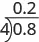Notice that the decimal point in the quotient is directly above the decimal point in the dividend.

To divide a decimal by a whole number, we place the decimal point in the quotient above the decimal point in the dividend and then divide as usual. Sometimes we need to use extra zeros at the end of the dividend to keep dividing until there is no remainder.

### How To

#### Divide a decimal by a whole number.

1. Step 1. Write as long division, placing the decimal point in the quotient above the decimal point in the dividend.
2. Step 2. Divide as usual.

### Example 5.19

Divide: $0.12÷3.0.12÷3.$

### Try It 5.37

Divide: $0.28÷4.0.28÷4.$

### Try It 5.38

Divide: $0.56÷7.0.56÷7.$

In everyday life, we divide whole numbers into decimals—money—to find the price of one item. For example, suppose a case of $2424$ water bottles cost $3.99.3.99.$ To find the price per water bottle, we would divide $3.993.99$ by $24,24,$ and round the answer to the nearest cent (hundredth).

### Example 5.20

Divide: $3.99÷24.3.99÷24.$

### Try It 5.39

Divide: $6.99÷36.6.99÷36.$

### Try It 5.40

Divide: $4.99÷12.4.99÷12.$

#### Divide a Decimal by Another Decimal

So far, we have divided a decimal by a whole number. What happens when we divide a decimal by another decimal? Let’s look at the same multiplication problem we looked at earlier, but in a different way.

$(0.2)(4)=0.8(0.2)(4)=0.8$

Remember, again, that a multiplication problem can be rephrased as a division problem. This time we ask, “How many times does $0.20.2$ go into $0.8?”0.8?”$ Because $(0.2)(4)=0.8,(0.2)(4)=0.8,$ we can say that $0.20.2$ goes into $0.80.8$ four times. This means that $0.80.8$ divided by $0.20.2$ is $4.4.$

$0.8÷0.2=40.8÷0.2=4$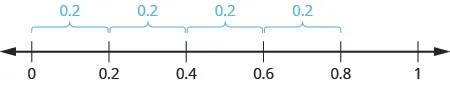We would get the same answer, $4,4,$ if we divide $88$ by $2,2,$ both whole numbers. Why is this so? Let’s think about the division problem as a fraction.

$0.80.2(0.8)10(0.2)108240.80.2(0.8)10(0.2)10824$

We multiplied the numerator and denominator by $1010$ and ended up just dividing $88$ by $4.4.$ To divide decimals, we multiply both the numerator and denominator by the same power of $1010$ to make the denominator a whole number. Because of the Equivalent Fractions Property, we haven’t changed the value of the fraction. The effect is to move the decimal points in the numerator and denominator the same number of places to the right.

We use the rules for dividing positive and negative numbers with decimals, too. When dividing signed decimals, first determine the sign of the quotient and then divide as if the numbers were both positive. Finally, write the quotient with the appropriate sign.

It may help to review the vocabulary for division: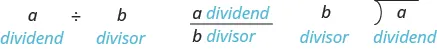### How To

#### Divide decimal numbers.

1. Step 1. Determine the sign of the quotient.
2. Step 2. Make the divisor a whole number by moving the decimal point all the way to the right. Move the decimal point in the dividend the same number of places to the right, writing zeros as needed.
3. Step 3. Divide. Place the decimal point in the quotient above the decimal point in the dividend.
4. Step 4. Write the quotient with the appropriate sign.

### Example 5.21

Divide: $−2.89÷(3.4).−2.89÷(3.4).$

### Try It 5.41

Divide: $−1.989÷5.1.−1.989÷5.1.$

### Try It 5.42

Divide: $−2.04÷5.1.−2.04÷5.1.$

### Example 5.22

Divide: $−25.65÷(−0.06).−25.65÷(−0.06).$

### Try It 5.43

Divide: $−23.492÷(−0.04).−23.492÷(−0.04).$

### Try It 5.44

Divide: $−4.11÷(−0.12).−4.11÷(−0.12).$

Now we will divide a whole number by a decimal number.

### Example 5.23

Divide: $4÷0.05.4÷0.05.$

### Try It 5.45

Divide: $6÷0.03.6÷0.03.$

### Try It 5.46

Divide: $7÷0.02.7÷0.02.$

### Use Decimals in Money Applications

We often apply decimals in real life, and most of the applications involving money. The Strategy for Applications we used in The Language of Algebra gives us a plan to follow to help find the answer. Take a moment to review that strategy now.

### Strategy for Applications

1. Identify what you are asked to find.
2. Write a phrase that gives the information to find it.
3. Translate the phrase to an expression.
4. Simplify the expression.
5. Answer the question with a complete sentence.

### Example 5.24

Paul received $5050$ for his birthday. He spent $31.6431.64$ on a video game. How much of Paul’s birthday money was left?

### Try It 5.47

Nicole earned $3535$ for babysitting her cousins, then went to the bookstore and spent $18.4818.48$ on books and coffee. How much of her babysitting money was left?

### Try It 5.48

Amber bought a pair of shoes for $24.7524.75$ and a purse for $36.90.36.90.$ The sales tax was $4.32.4.32.$ How much did Amber spend?

### Example 5.25

Jessie put $88$ gallons of gas in her car. One gallon of gas costs $3.529.3.529.$ How much does Jessie owe for the gas? (Round the answer to the nearest cent.)

### Try It 5.49

Hector put $1313$ gallons of gas into his car. One gallon of gas costs $3.175.3.175.$ How much did Hector owe for the gas? Round to the nearest cent.

### Try It 5.50

Christopher bought $55$ pizzas for the team. Each pizza cost $9.75.9.75.$ How much did all the pizzas cost?

### Example 5.26

Four friends went out for dinner. They shared a large pizza and a pitcher of soda. The total cost of their dinner was $31.76.31.76.$ If they divide the cost equally, how much should each friend pay?

### Try It 5.51

Six friends went out for dinner. The total cost of their dinner was $92.82.92.82.$ If they divide the bill equally, how much should each friend pay?

### Try It 5.52

Chad worked $4040$ hours last week and his paycheck was $570.570.$ How much does he earn per hour?

Be careful to follow the order of operations in the next example. Remember to multiply before you add.

### Example 5.27

Marla buys $66$ bananas that cost $0.220.22$ each and $44$ oranges that cost $0.490.49$ each. How much is the total cost of the fruit?

### Try It 5.53

Suzanne buys $33$ cans of beans that cost $0.750.75$ each and $66$ cans of corn that cost $0.620.62$ each. How much is the total cost of these groceries?

### Try It 5.54

Lydia bought movie tickets for the family. She bought two adult tickets for $9.509.50$ each and four children’s tickets for $6.006.00$ each. How much did the tickets cost Lydia in all?

### Section 5.2 Exercises

#### Practice Makes Perfect

In the following exercises, add or subtract.

95.

$16.92 + 7.56 16.92 + 7.56$

96.

$18.37 + 9.36 18.37 + 9.36$

97.

$256.37 − 85.49 256.37 − 85.49$

98.

$248.25 − 91.29 248.25 − 91.29$

99.

$21.76 − 30.99 21.76 − 30.99$

100.

$15.35 − 20.88 15.35 − 20.88$

101.

$37.5 + 12.23 37.5 + 12.23$

102.

$38.6 + 13.67 38.6 + 13.67$

103.

$− 16.53 − 24.38 − 16.53 − 24.38$

104.

$− 19.47 − 32.58 − 19.47 − 32.58$

105.

$− 38.69 + 31.47 − 38.69 + 31.47$

106.

$− 29.83 + 19.76 − 29.83 + 19.76$

107.

$− 4.2 + ( − 9.3 ) − 4.2 + ( − 9.3 )$

108.

$− 8.6 + ( − 8.6 ) − 8.6 + ( − 8.6 )$

109.

$100 − 64.2 100 − 64.2$

110.

$100 − 65.83 100 − 65.83$

111.

$72.5 − 100 72.5 − 100$

112.

$86.2 − 100 86.2 − 100$

113.

$15 + 0.73 15 + 0.73$

114.

$27 + 0.87 27 + 0.87$

115.

$2.51 + 40 2.51 + 40$

116.

$9.38 + 60 9.38 + 60$

117.

$91.75 − ( − 10.462 ) 91.75 − ( − 10.462 )$

118.

$94.69 − ( − 12.678 ) 94.69 − ( − 12.678 )$

119.

$55.01 − 3.7 55.01 − 3.7$

120.

$59.08 − 4.6 59.08 − 4.6$

121.

$2.51 − 7.4 2.51 − 7.4$

122.

$3.84 − 6.1 3.84 − 6.1$

Multiply Decimals

In the following exercises, multiply.

123.

$( 0.3 ) ( 0.4 ) ( 0.3 ) ( 0.4 )$

124.

$( 0.6 ) ( 0.7 ) ( 0.6 ) ( 0.7 )$

125.

$( 0.24 ) ( 0.6 ) ( 0.24 ) ( 0.6 )$

126.

$( 0.81 ) ( 0.3 ) ( 0.81 ) ( 0.3 )$

127.

$( 5.9 ) ( 7.12 ) ( 5.9 ) ( 7.12 )$

128.

$( 2.3 ) ( 9.41 ) ( 2.3 ) ( 9.41 )$

129.

$( 8.52 ) ( 3.14 ) ( 8.52 ) ( 3.14 )$

130.

$( 5.32 ) ( 4.86 ) ( 5.32 ) ( 4.86 )$

131.

$(−4.3)(2.71) (−4.3)(2.71)$

132.

$( − 8.5 ) ( 1.69 ) ( − 8.5 ) ( 1.69 )$

133.

$(−5.18)(−65.23) (−5.18)(−65.23)$

134.

$( − 9.16 ) ( − 68.34 ) ( − 9.16 ) ( − 68.34 )$

135.

$( 0.09 ) ( 24.78 ) ( 0.09 ) ( 24.78 )$

136.

$( 0.04 ) ( 36.89 ) ( 0.04 ) ( 36.89 )$

137.

$( 0.06 ) ( 21.75 ) ( 0.06 ) ( 21.75 )$

138.

$( 0.08 ) ( 52.45 ) ( 0.08 ) ( 52.45 )$

139.

$( 9.24 ) ( 10 ) ( 9.24 ) ( 10 )$

140.

$( 6.531 ) ( 10 ) ( 6.531 ) ( 10 )$

141.

$( 55.2 ) ( 1,000 ) ( 55.2 ) ( 1,000 )$

142.

$( 99.4 ) ( 1,000 ) ( 99.4 ) ( 1,000 )$

Divide Decimals

In the following exercises, divide.

143.

$0.15 ÷ 5 0.15 ÷ 5$

144.

$0.27 ÷ 3 0.27 ÷ 3$

145.

$4.75 ÷ 25 4.75 ÷ 25$

146.

$12.04 ÷ 43 12.04 ÷ 43$

147.

$8.49 ÷ 12 8.49 ÷ 12$

148.

$16.99 ÷ 9 16.99 ÷ 9$

149.

$117.25 ÷ 48 117.25 ÷ 48$

150.

$109.24 ÷ 36 109.24 ÷ 36$

151.

$0.6 ÷ 0.2 0.6 ÷ 0.2$

152.

$0.8 ÷ 0.4 0.8 ÷ 0.4$

153.

$1.44 ÷ ( − 0.3 ) 1.44 ÷ ( − 0.3 )$

154.

$1.25 ÷ ( − 0.5 ) 1.25 ÷ ( − 0.5 )$

155.

$− 1.75 ÷ ( − 0.05 ) − 1.75 ÷ ( − 0.05 )$

156.

$− 1.15 ÷ ( − 0.05 ) − 1.15 ÷ ( − 0.05 )$

157.

$5.2 ÷ 2.5 5.2 ÷ 2.5$

158.

$6.5 ÷ 3.25 6.5 ÷ 3.25$

159.

$12 ÷ 0.08 12 ÷ 0.08$

160.

$5 ÷ 0.04 5 ÷ 0.04$

161.

$11 ÷ 0.55 11 ÷ 0.55$

162.

$14 ÷ 0.35 14 ÷ 0.35$

Mixed Practice

In the following exercises, simplify.

163.

$6 ( 12.4 − 9.2 ) 6 ( 12.4 − 9.2 )$

164.

$3 ( 15.7 − 8.6 ) 3 ( 15.7 − 8.6 )$

165.

$24 ( 0.5 ) + ( 0.3 ) 2 24 ( 0.5 ) + ( 0.3 ) 2$

166.

$35 ( 0.2 ) + ( 0.9 ) 2 35 ( 0.2 ) + ( 0.9 ) 2$

167.

$1.15 ( 26.83 + 1.61 ) 1.15 ( 26.83 + 1.61 )$

168.

$1.18 ( 46.22 + 3.71 ) 1.18 ( 46.22 + 3.71 )$

169.

$45 + 0.08 ( 45 ) 45 + 0.08 ( 45 )$

170.

$63 + 0.18 ( 63 ) 63 + 0.18 ( 63 )$

171.

$18 ÷ ( 0.75 + 0.15 ) 18 ÷ ( 0.75 + 0.15 )$

172.

$27 ÷ ( 0.55 + 0.35 ) 27 ÷ ( 0.55 + 0.35 )$

173.

$( 1.43 + 0.27 ) ÷ ( 0.9 − 0.05 ) ( 1.43 + 0.27 ) ÷ ( 0.9 − 0.05 )$

174.

$( 1.5 − 0.06 ) ÷ ( 0.12 + 0.24 ) ( 1.5 − 0.06 ) ÷ ( 0.12 + 0.24 )$

175.

$[ 75.42 + 0.18 ( 75.42 ) ] ÷ 5 [ 75.42 + 0.18 ( 75.42 ) ] ÷ 5$

176.

$[ 56.31 + 0.22 ( 56.31 ) ] ÷ 4 [ 56.31 + 0.22 ( 56.31 ) ] ÷ 4$

Use Decimals in Money Applications

In the following exercises, use the strategy for applications to solve.

177.

Spending money Brenda got $4040$ from the ATM. She spent $15.1115.11$ on a pair of earrings. How much money did she have left?

178.

Spending money Marissa found $2020$ in her pocket. She spent $4.824.82$ on a smoothie. How much of the $2020$ did she have left?

179.

Shopping Adam bought a t-shirt for $18.4918.49$ and a book for $8.928.92$ The sales tax was $1.65.1.65.$ How much did Adam spend?

180.

Restaurant Roberto’s restaurant bill was $20.4520.45$ for the entrée and $3.153.15$ for the drink. He left a $4.404.40$ tip. How much did Roberto spend?

181.

Coupon Emily bought a box of cereal that cost $4.29.4.29.$ She had a coupon for $0.550.55$ off, and the store doubled the coupon. How much did she pay for the box of cereal?

182.

Coupon Diana bought a can of coffee that cost $7.99.7.99.$ She had a coupon for $0.750.75$ off, and the store doubled the coupon. How much did she pay for the can of coffee?

183.

Diet Leo took part in a diet program. He weighed $190190$ pounds at the start of the program. During the first week, he lost $4.34.3$ pounds. During the second week, he had lost $2.82.8$ pounds. The third week, he gained $0.70.7$ pounds. The fourth week, he lost $1.91.9$ pounds. What did Leo weigh at the end of the fourth week?

184.

Snowpack On April $1,1,$ the snowpack at the ski resort was $44$ meters deep, but the next few days were very warm. By April $5,5,$ the snow depth was $1.61.6$ meters less. On April $8,8,$ it snowed and added $2.12.1$ meters of snow. What was the total depth of the snow?

185.

Coffee Noriko bought $44$ coffees for herself and her co-workers. Each coffee was $3.75.3.75.$ How much did she pay for all the coffees?

186.

Subway Fare Arianna spends $4.504.50$ per day on subway fare. Last week she rode the subway $66$ days. How much did she spend for the subway fares?

187.

Income Mayra earns $9.259.25$ per hour. Last week she worked $3232$ hours. How much did she earn?

188.

Income Peter earns $8.758.75$ per hour. Last week he worked $1919$ hours. How much did he earn?

189.

Hourly Wage Alan got his first paycheck from his new job. He worked $3030$ hours and earned $382.50.382.50.$ How much does he earn per hour?

190.

Hourly Wage Maria got her first paycheck from her new job. She worked $2525$ hours and earned $362.50.362.50.$ How much does she earn per hour?

191.

Restaurant Jeannette and her friends love to order mud pie at their favorite restaurant. They always share just one piece of pie among themselves. With tax and tip, the total cost is $6.00.6.00.$ How much does each girl pay if the total number sharing the mud pie is

$2 ? 2 ?$

$3 ? 3 ?$

$4 ? 4 ?$

$5 ? 5 ?$

$6 ? 6 ?$

192.

Pizza Alex and his friends go out for pizza and video games once a week. They share the cost of a $15.6015.60$ pizza equally. How much does each person pay if the total number sharing the pizza is

$2 ? 2 ?$

$3 ? 3 ?$

$4 ? 4 ?$

$5 ? 5 ?$

$6 ? 6 ?$

193.

Fast Food At their favorite fast food restaurant, the Carlson family orders $44$ burgers that cost $3.293.29$ each and $22$ orders of fries at $2.742.74$ each. What is the total cost of the order?

194.

Home Goods Chelsea needs towels to take with her to college. She buys $22$ bath towels that cost $9.999.99$ each and $66$ washcloths that cost $2.992.99$ each. What is the total cost for the bath towels and washcloths?

195.

Zoo The Lewis and Chousmith families are planning to go to the zoo together. Adult tickets cost $29.9529.95$ and children’s tickets cost $19.95.19.95.$ What will the total cost be for $44$ adults and $77$ children?

196.

Ice Skating Jasmine wants to have her birthday party at the local ice skating rink. It will cost $8.258.25$ per child and $12.9512.95$ per adult. What will the total cost be for $1212$ children and $33$ adults?

#### Everyday Math

197.

Paycheck Annie has two jobs. She gets paid $14.0414.04$ per hour for tutoring at City College and $8.758.75$ per hour at a coffee shop. Last week she tutored for $88$ hours and worked at the coffee shop for $1515$ hours.

How much did she earn?

If she had worked all $2323$ hours as a tutor instead of working both jobs, how much more would she have earned?

198.

Paycheck Jake has two jobs. He gets paid $7.957.95$ per hour at the college cafeteria and $20.2520.25$ at the art gallery. Last week he worked $1212$ hours at the cafeteria and $55$ hours at the art gallery.

How much did he earn?

If he had worked all $1717$ hours at the art gallery instead of working both jobs, how much more would he have earned?

#### Writing Exercises

199.

In the 2010 winter Olympics, two skiers took the silver and bronze medals in the Men's Super-G ski event. The silver medalist's time was $11$ minute $30.6230.62$ seconds and bronze medalist's time was $11$ minute $30.6530.65$ seconds. Whose time was faster? Find the difference in their times and then write the name of that decimal.

200.

Find the quotient of $0.12÷0.040.12÷0.04$ and explain in words all the steps taken.

#### Self Check

After completing the exercises, use this checklist to evaluate your mastery of the objectives of this section.

After reviewing this checklist, what will you do to become confident for all objectives?

Order a print copy

As an Amazon Associate we earn from qualifying purchases.